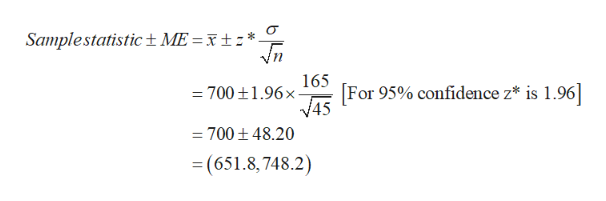# A sample of 45 small generators produce fumes that was observed to be 700 ppm with a standard deviation of 165. a) Calculate and interpret 95% two-sided confidence interval for true average amount of fumes for a population of all similar generatorsb) Assume the intial observations were based on an initial estimate of 170 for the value of the sample standard deviation. What is the necessary sample size that would result in an interval width of 40 ppm for a confidence level of 95% Show all work

Question

A sample of 45 small generators produce fumes that was observed to be 700 ppm with a standard deviation of 165.
a) Calculate and interpret 95% two-sided confidence interval for true average amount of fumes for a population of all similar generators
b) Assume the intial observations were based on an initial estimate of 170 for the value of the sample standard deviation. What is the necessary sample size that would result in an interval width of 40 ppm for a confidence level of 95%

Show all work

check_circleExpert Solution
Step 1

(a)

The 95% confidence interval for true average amount of fumes for a population of all similar generators is obtained as follows:help_outlineImage TranscriptioncloseSamplestatistic+ ME =xt * 165 For 95% confidence z* is 1.96 45 =700t1.96x = 700 t 48.20 (651.8,748.2) fullscreen
Step 2

Thus, the 95% confidence interval for true average amount of fumes for a population of all similar generators is (651.8, 748.2).

Interpretation:

There is 95% confidence that the true a...

### Want to see the full answer?

See Solution

#### Want to see this answer and more?

Solutions are written by subject experts who are available 24/7. Questions are typically answered within 1 hour*

See Solution
*Response times may vary by subject and question
Tagged in

### Hypothesis Testing